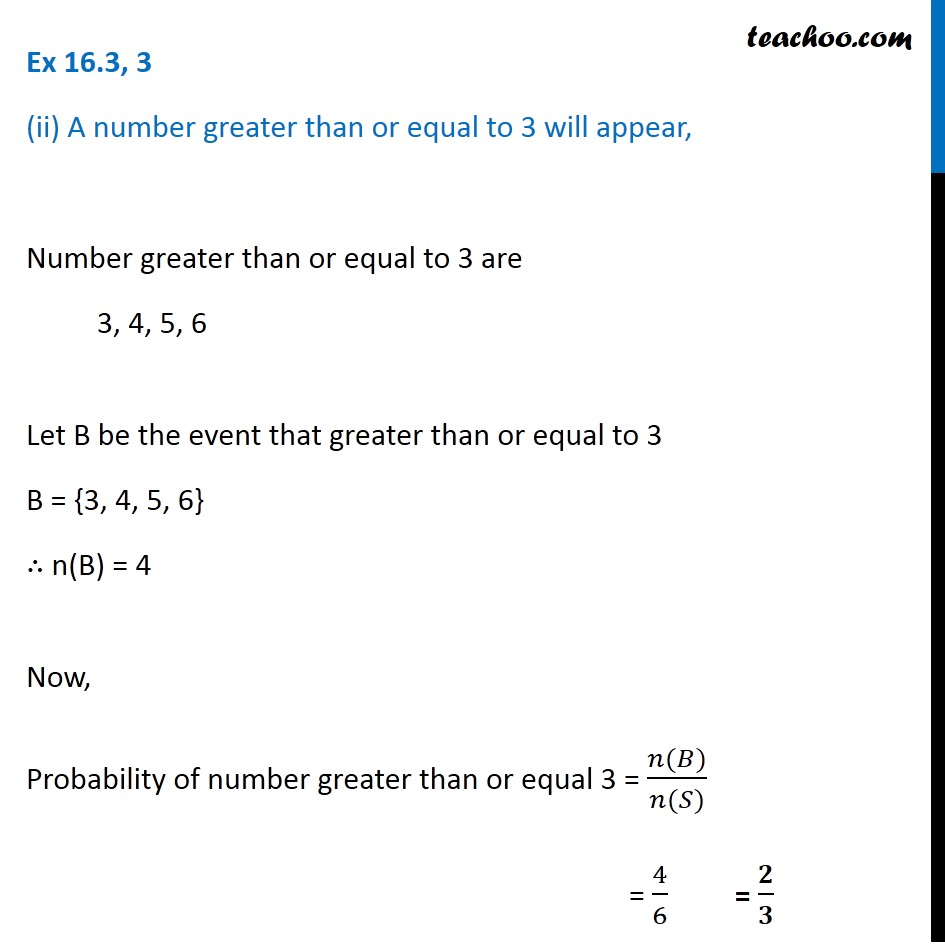Ex 16.3

Chapter 16 Class 11 Probability
Serial order wiseGet live Maths 1-on-1 Classs - Class 6 to 12

### Transcript

Ex 16.3, 3 (ii) A number greater than or equal to 3 will appear, Number greater than or equal to 3 are 3, 4, 5, 6 Let B be the event that greater than or equal to 3 B = {3, 4, 5, 6} ∴ n(B) = 4 Now, Probability of number greater than or equal 3 = (𝑛(𝐵))/(𝑛(𝑆)) = 4/6 = 𝟐/𝟑